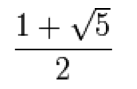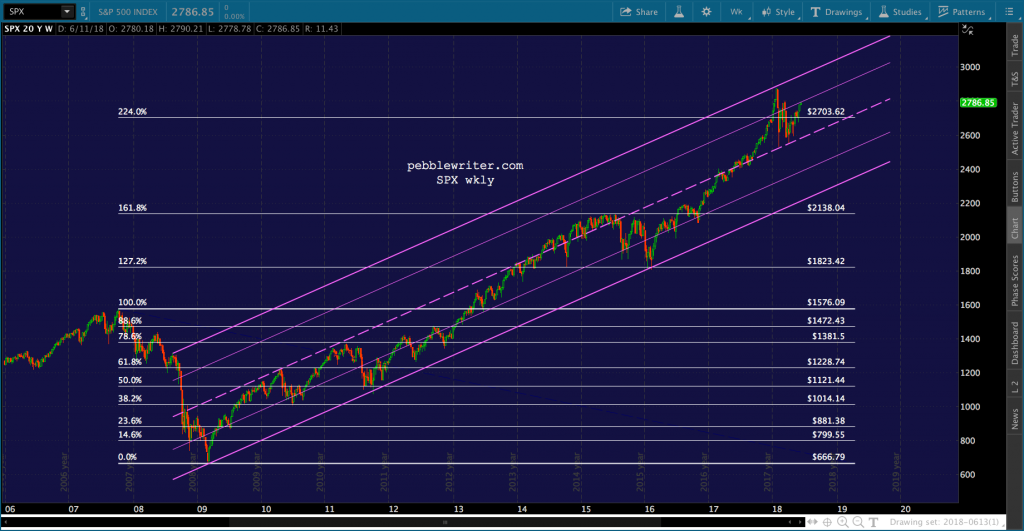# fibonacci

The importance of Fibonacci numbers and ratios is a great mystery.  They relate to a great many phenomena in both the natural (plant and flower design, the family trees of honeybees, shells, etc.) and the man-made world (the layout of Egyptian pyramids, the dimensions of the Parthenon, modern-day fashion and design).Perhaps the greatest mathematician of the Middle Ages, Leonardo of Pisa (aka Leonardo Fibonacci), wrote The Liber Abaci in 1202.  In it, he not only popularized the use of Hindu-Arabic numerals over more cumbersome Roman numerals, but also cited a special series of numbers previously little known outside India — the Fibonacci Series:The series is formed by adding the sum of two previous numbers to obtain a third.  By convention, the series begins with “0” and “1” and yields the following set: {(0+1=1), (1+1=2), (1+2=3), (2+3=5), (5+3=8)…}, or  {0, 1, 1, 2, 3, 5, 8, 13, 21, 34, 55, 89, 144…}.

As the numbers get larger, the relationship of each number to the number below it approaches the Golden Ratio…which equals 1.61803398874989, or as it’s commonly rounded, 1.618.  The relationship of each number to the number above approaches the inverse, or .618.

These two numbers are at the core of a set of Fibonacci ratios that are commonly used in investing.

.146 — .6184

.236 — .6183

.382 — .6182

.500 — Fib 1/Fib 2

.618 — inverse of Golden Ratio

.707 — .500.5

.786 — .618.5

.886 — .618.25

1.000 — any Fib number div by itself

1.272 — 1.618.5

1.618 — Golden Ratio

2.000 — Fib 2/Fib 1

2.24 — 5.5

2.618 — 1.6182

3.618 — 1.6182

4.236 — 1.6183

6.854 — 1.6184

11.090 — 1.6185

17.944 — 1.6186

29.034 — 1.6187

46.979 — 1.6188

76.013 — 1.6189

122.992 — 1.61810

For investment purposes, Fibonacci ratios matter because they very often relate to the degree to which price action retraces a previous move.  A decline of X, for instance, is often followed by a retracement (a bounce) of .5, .618, .786 or .886 of X.  After such a retracement, the next primary move forward is often 1.618 times the original X (an extension versus a retracement).

Following the Great Depression, for instance, significant reversals were seen not only at the 1.618 extension of the original 1929-1932 decline, but also at 2.000, 2.618, 3.618 and 4.236.In recent years, we have seen significant reversals take place at major Fibonacci retracement and extension levels.  Note the sizeable drops at the .618, 786 and .886 Fib retracements and the 1.618 Fib extension.  Most significant drops terminated at a lower Fib level, with the most striking one being the 1.272 extension at 1823.  After being ignored as overhead resistance, it served as support multiple times.Importantly, Fibonacci Ratios relate to time as well as price.  That is, the amount of time between important price movements frequently relate to one another as Fibonacci Ratios — both retracements and extensions.  Lately, my work is increasingly focused on combining Fibonacci price and time movements.Because Fibonacci numbers/ratios all stem from the same series, it’s not surprising that they can overlap when viewed on different scales.  The .618 of one set can be the 1.618 of another set, as seen on the following Fibonacci time ratio chart.Fibonacci ratios have been studied in quite some detail over the years, yielding a set of frequently seen combinations of retracements and extensions known as Harmonics Patterns.  The most common of these patterns, shown below, are explored in more detail elsewhere in this section.